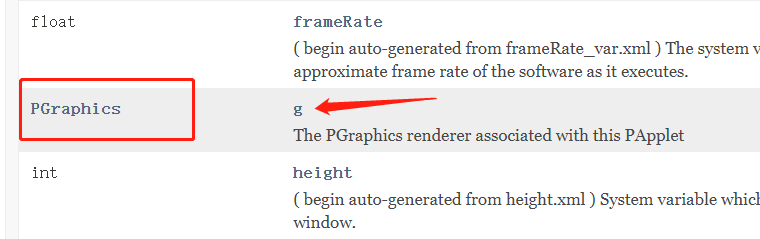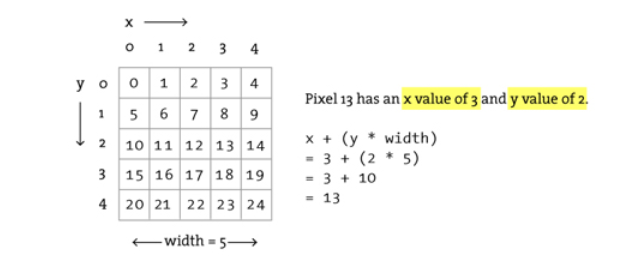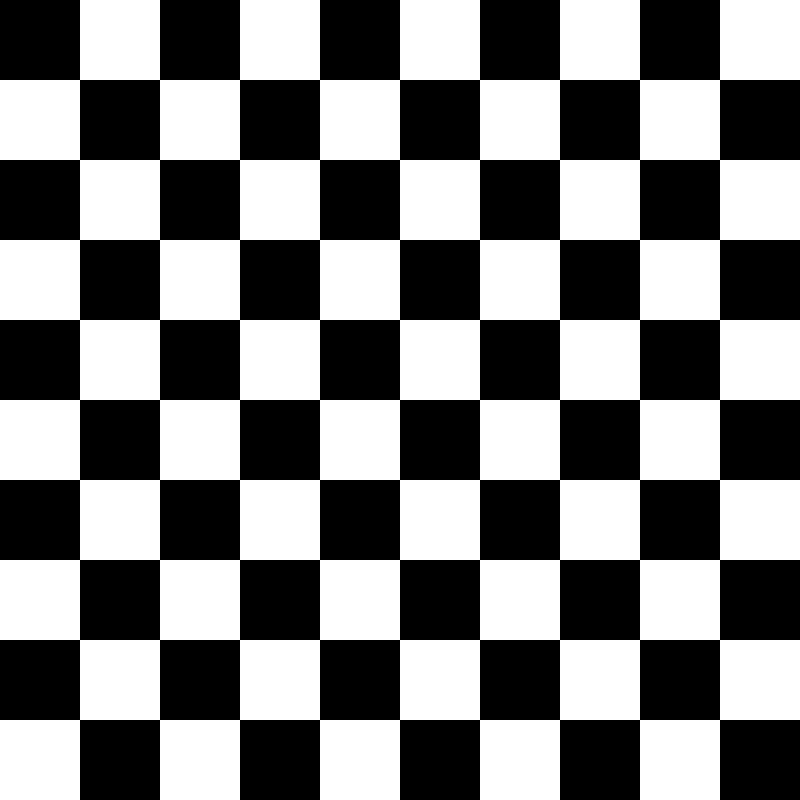# 第一步

``````void setup() {
size(400, 400);

pixels = color(0);
updatePixels();

}
````````````void setup() {
size(400, 400);  //定义的该PApplet对于surface视口大小,
//并且也创建了一个内置PGraphics，命名为g，大小是（width,height）,并做了初识化
g.pixels = color(0);
g.updatePixels();

}
``````

``````PGraphics mygraphics;
void setup() {
size(400, 400);  //定义的该PApplet对于surface视口大小
mygraphics = createGraphics(width,height);//创建graphics,大小为width，height,画布大小
mygraphics.beginDraw();                   //这两行实则在做PGraphics的pixels[]等属性的初始化，
mygraphics.endDraw();                     //如果不加，下面的pixels就获取不到，会抛空指针异常

mygraphics.pixels = color(0);
mygraphics.updatePixels();

}
``````

# 言归正传``````for (int x = 0; x < width; x++) {
for (int y = 0; y < height; y++) {
int loc = x + y * width;      //这是一个公式，以横纵两轴向进行遍历，一位数组带被映射到二维数组中的某个值，即图中的某个像素点，可用loc = x + y *width来表示
pixels[loc] = color(0);     //那么loc 这个索引号 代表的是 （x,y） 这一个像素点
}
}
``````

``````  increW = width/WCOUNT;
increH = height/HCOUNT;
``````

``````int k= 0;
void draw()
{
k ++;
if(k % 2 == 0)
pixels[x + y * width] = color(0);
if(k % 2 != 0)
pixels[x + y * width] = color(255);
}
``````

``````indexH ++ ;        //这个东东是什么？又来了个增幅？还是开关变量？其实它才是真正控制步长的变量。
if (indexH % increH == 0)//注意表达式，同样做求余运算，判断是否取到0，如果是，则刚好index加了一个步长
{
indexH = 0;      //当到了一个步长，那步长计数要归零，重新计数(其实这里可以省略，因为求余算法可以弥补，但是为了设计算法方便，可以保留)
k ++;
}
``````

``````  increW = width/WCOUNT;
increH = height/HCOUNT;
int k = 0;
int indexH = 0;
int indexW = 0;
for (int x = 0; x  < width; x ++)
{
for (int y = 0; y < height; y ++)
{
if (k % 2 == 0)
{
pixels[x + y * width] = color(0);
} else
{
pixels[x + y * width] = color(255);
}
///////第一层逻辑控制，控制纵向绘制时每走步长数换一次K//////////
indexH ++ ;
if (indexH % increH == 0)
{
indexH = 0;
k ++;
}
///////第一层逻辑控制//////////
}
///////第二层逻辑控制，控制横向绘制时每走步长数换一次K//////////
indexW ++ ;
if (indexW % increW == 0)
{
indexW = 0;
k ++;
}
///////第二层逻辑控制//////////

}
``````

``````int increW;
int increH;
int WCOUNT = 10;
int HCOUNT = 10;

void settings() {
size(800, 800);
}
void setup() {
increW = width/WCOUNT;
increH = height/HCOUNT;
int k = 0;
int indexH = 0;
int indexW = 0;
for (int x = 0; x  < width; x ++)
{
for (int y = 0; y < height; y ++)
{
if (k % 2 == 0)
{
pixels[x + y * width] = color(0);
} else
{
pixels[x + y * width] = color(255);
}
indexH ++ ;
if (indexH % increH == 0)
{
indexH = 0;
k ++;
}
}
indexW ++ ;
if (indexW % increW == 0)
{
indexW = 0;
k ++;
}
}
updatePixels();
}

void draw() {
}

``````

# 言外

``````int increW;
int increH;
int WCOUNT = 10;
int HCOUNT = 10;

void settings() {
size(800, 800);
}
void setup() {
increW = width/WCOUNT;
increH = height/HCOUNT;
int k = 0;
int indexH = 0;
int indexW = 0;
for (int x = 0; x  < width; x ++)
{
for (int y = 0; y < height; y ++)
{
if (k % 2 == 0)
{
set(x, y, color(0));
} else
{
set(x, y, color(255));
}
indexH ++ ;
if (indexH % increH == 0)
k ++;
}
indexW ++ ;
if (indexW % increH == 0)
k ++;
}
//updatePixels();
}

void draw() {
}

``````

# 结果